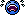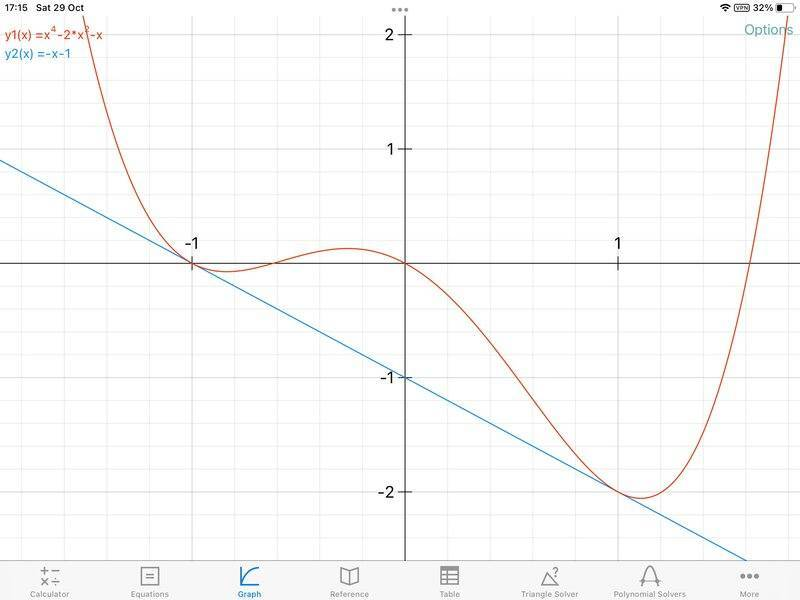# Find the two points on the curve that share a tangent line

• Graxum
... factoring out ##(a+b)## from the equation.##\displaystyle (b^2 + a^2 - 2 + 4ab)(a+b)##will only have a positive value if ##\displaystyle a## is greater than ##\displaystyle b##.f

#### Graxum

Homework Statement
Find the two points on the curve y=x^4 - 2x^2 - x that share a tangent line?
Relevant Equations
y=x^4 - 2x^2 - x

dy/dx = 4x^3 - 4x - 1
IMPORTANT: NO CALCULATORS

I assumed two points, (a, f(a)) and (b, f(b)) where b is greater than a. Since the tangent line is shared, I did

f'(a) = f'(b):

1) 4a^3 - 4a - 1 = 4b^3 - 4b - 1
2) 4a^3 - 4a = 4b^3 - 4b
3) 4(a^3 - a) = 4(b^3 - b)
4) a^3 - a = b^3 - b
5) a^3 - b^3 = a - b
6) (a - b)(a^2 + ab + b^2) = (a - b)
7) a^2 + ab + b^2 = 1

and

(f(b) - f(a))/b - a = f'(a):

1) (b^4 - 2b^2 - b - a^4 + 2a^2 + a) / (b - a) = 4a^3 - 4a - 1
2) [(b^4 - a^4) -2(b^2 - a^2) - (b - a)] / (b - a) = 4a^3 -4a -1
3) [(b^2 + a^2)(b + a)(b - a) - 2(b + a)(b - a) - (b - a)] / (b - a) = 4a^3 - 4a - 1
4) (b^2 + a^2)(b + a) - 2(b + a) - 1 = 4a^3 - 4a - 1
5) (b + a)(b^2 + a^2 - 2) = 4a(a - 1)(a + 1)
6) From the 7th step of the 1st equation, I deduced that:
a^2 + b(a + b) = 1
6.1) b(a + b) = (1 - a)(1 + a), thus -b(a + b) = (a - 1)(a + 1)
7) Subbing in -b(a + b) for (a - 1)(a + 1) on the right side of the equation on the 5th line, I got:
(b + a)(b^2 + a^2 - 2) = -4ab(a + b)
8) b^2 + a^2 - 2 = -4ab
9) From the 7th step of the 1st equation, I deduced that:
a^2 + b^2 = 1 - ab
10) Subbing in 1 - ab for a^2 + b^2 on the left side of the equation on the 8th line, I got:
-ab - 1 = -4ab
11) 1 = 3ab, thus ab = 1/3
12) Subbing in ab for 1 - a^2 - b^2 on the right side of the equation on the 8th line, I got:
a^2 + b^2 - 2 = -4 + 4a^2 + 4b^2
13) a^2 + b^2 = 2/3

Now you would think that knowing ab = 1/3 and a^2 + b^2 = 2/3 would make things easy, however I must've made a mistake somewhere because I find multiple possible solutions, a=1 (and f(a) for the y coordinate) and 2 values for b (those being 2/3 or -1, and their respective y coordinates.) I came so far and still can't find the answer!

Homework Statement:: Find the two points on the curve y=x^4 - 2x^2 - x that share a tangent line?
Relevant Equations:: y=x^4 - 2x^2 - x

dy/dx = 4x^3 - 4x - 1

IMPORTANT: NO CALCULATORS

I assumed two points, (a, f(a)) and (b, f(b)) where b is greater than a. Since the tangent line is shared, I did

f'(a) = f'(b):
...

and

(f(b) - f(a))/b - a = f'(a):
...

7) Subbing in -b(a + b) for (a - 1)(a + 1) on the right side of the equation on the 5th line, I got:
(b + a)(b^2 + a^2 - 2) = -4ab(a + b)
8) b^2 + a^2 - 2 = -4ab
...

Now you would think that knowing ab = 1/3 and a^2 + b^2 = 2/3 would make things easy, however I must've made a mistake somewhere because I find multiple possible solutions, a=1 (and f(a) for the y coordinate) and 2 values for b (those being 2/3 or -1, and their respective y coordinates.) I came so far and still can't find the answer!
Hello, @Graxum .Be careful going from the result of step 7) to step 8).

You divided by ##(a+b)## . That is not legal if the value of ##(a+b)## is zero.

It's better to get zero on one side of the equation, then factor out ##(a+b)##.

That should also give the possibility that ##(a+b)=0##, in addition to the result you obtained.

At this point the problem is nearly solved. (Being a bit redundant, let's continue.)

##\displaystyle (b^2 + a^2 - 2 + 4ab)(a+b)=0##

So one of the two factors, ##\displaystyle (b^2 + a^2 - 2 + 4ab)## or ##\displaystyle (a+b)## must be zero.

You have already tried setting the first of these to zero. Now try the other, along with the 7th step of the 1st equation.

Last edited:
•Graxum
Hint:
$$\frac{dy}{dx}=\frac{y}{x}$$

Hint:
$$\frac{dy}{dx}=\frac{y}{x}$$
That doesn't seem to me to be correct.

(f(b) - f(a))/b - a = f'(a):
I think keeping the problem symmetric in ##a## and ##b## will significantly simplify your algebra. Try writing your equation for the shared tangent line as:$$\frac{f(b)-f(a)}{b-a}=\frac{f^{\prime}(a)+f^{\prime}(b)}{2}$$ and repeating your derivation.

Let the tangent line be $y = mx + c$, and define $$g(x) = f(x) - mx - c = x^4 - 2x^2 - (m+1)x - c.$$ Npw if $(a, f(a))$ is on the tangent line then $g(a) = 0$, and for the line to actually be a tangent we require $g'(a) = 0$. We obtain the same conditions for $b$. Can you see why the fact that $g$ is a polynomial of order 4 in $x$ then requires $$g(x) = (x- a)^2(x- b)^2 = x^4 - 2x^2 - (m+1)x - c?$$ Comparing coefficients of $x^3$ and $x^2$ will now give you an easier system of equations to solve for $a$ and $b$.

•SammyS
Hello, @Graxum .Be careful going from the result of step 7) to step 8).

You divided by ##(a+b)## . That is not legal if the value of ##(a+b)## is zero.

It's better to get zero on one side of the equation, then factor out ##(a+b)##.

That should also give the possibility that ##(a+b)=0##, in addition to the result you obtained.

At this point the problem is nearly solved. (Being a bit redundant, let's continue.)

##\displaystyle (b^2 + a^2 - 2 + 4ab)(a+b)=0##

So one of the two factors, ##\displaystyle (b^2 + a^2 - 2 + 4ab)## or ##\displaystyle (a+b)## must be zero.

You have already tried setting the first of these to zero. Now try the other, along with the 7th step of the 1st equation.
Ah i completely forgot about that!

I have 1 more question though, After the correction I got 2 answers ##(a= -1/sqrt3, b= 1/sqrt3)## from the left side and ##(a=-1, b=1)## from the right side, how do I check which of those is the correct answer? are both correct? in that case, when I plot the main equation on a graph, the 1st one seems to be completely off and those points definitely don't share a tangent line.

I wonder if I calculated something wrong somewhere?

Ah i completely forgot about that!

I have 1 more question though, After the correction I got 2 answers ##(a= -1/\sqrt 3, b= 1/\sqrt 3)## from the left side and ##(a=-1, b=1)## from the right side, how do I check which of those is the correct answer? are both correct? in that case, when I plot the main equation on a graph, the 1st one seems to be completely off and those points definitely don't share a tangent line.

I wonder if I calculated something wrong somewhere?
Well, you could plug those values into check them.

Beyond that:
For the following equation to be true,

##\displaystyle (b^2 + a^2 - 2 + 4ab)(a+b)=0##

all that's required is that either ##\displaystyle (b^2 + a^2 - 2 + 4ab)=0 \text{ or } (a+b)=0##

It looks like you found that ##a+b=0## leads to ##a=-1, \ b=1## .

That does give the correct solution.

I thought I was fairly well up in polynomials, for an amateur, and that this would be a doddle. Instead I found it quite difficult, maybe the operative word there is 'was'..

I didn't go through the OP's calculations, they looked too heavy and complicated. When it gets that complicated one should look for a simpler approach.

But then I realize that is even when true easier said than done sometimes. especially if you had a method which you are sure should work, so if it doesn't you might think there is some calculational error and ask for help as the OP did.

I know how to find the condition for a double root of a polynomial, I do not know a formulation for finding two double roots any more compact than finding one and then the other.

Anyway I eventually found (independently) essentially the same formulation as that of pasmith, and it all falls out in a couple of lines, with result ##m = -1, c=-1##. You do not even need to consider the derivative. So I can only add, seeing is believing:I'll worry about how general this is some other time.

Last edited: